# Isoperimetric problem, Steiner symmetrization and Edler's proof — how many applications does a paper clip have?I am reminded of the question, which was asked to pre-school children "How many uses do you think of a paper clip?". The query was repeated on the same group after several years. The conclusions were simple — the older we are, the smaller the chance that we will think about a paper clip as a meat skewer or about the Steiner symmetrization as a fly-flap. But more on that later, coming back to the topic — Steiner symmetrization and Edler's proof!

# A bit of history

The determination of maximum and minimum quantities of given objects (figures, functions, shapes) has been interesting for scientists for a long time. In Antiquity it was known that the isosceles triangle has a larger area than any other triangle with the same base and the same perimeter, and that among the polygons with a fixed perimeter and the same number of sides, the regular polygon has the largest area. The author of these claims is Zenodor (3rd and 2nd century BC). Issues of this kind have departed from the horizon of scholars for many centuries, the change took place in the seventeenth century as a result of the discovery of the differential calculus and later of the variational calculus. New tools have enabled mathematicians to consider the problem in a different way and solve isoperimetric problems on the way that gives the geometric approach a wide berth. But even here there were exceptions — Gabriel Cramer, Simon Antoine Jean L'Huillier and Jacob Steiner were still working on solutions derived from elementary knowledge. The latter has become famous for solving the isoperimetric problem — the answer to the question: why the circle has the largest area among the curves with a fixed perimeter?

# Isoperimetric problem

Let's follow Steiner and his reasoning. Here we omit the existential part of the proof temporarily — we assume that the solution exists, so the searched curve K has the length L and the maximum area. The first step is to eliminate the closed concave curves, the curve K have to be convex, ie. the section connecting any two points of the curve K must lie completely inside or be included in the curve K. Otherwise — it would be possible to lead the section lying outside the curve K. Let us consider why curve K have to be convex.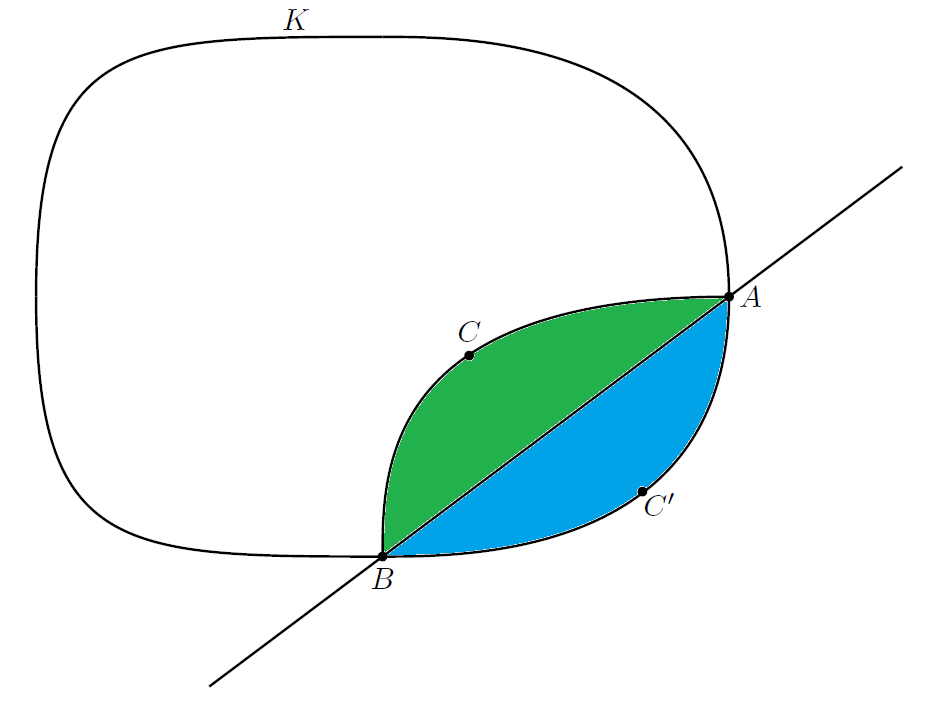Concave curve sucks.

The above figure shows a concave curve (containing a C point) and a convex curve (including a C' point). The AC'B curve is so arranged that its length is equal to the ACB curve. As a result, the concave and convex curves have equal perimeters, and with the naked eye it can be seen that the convex curve covers a larger area than a concave one (which is smaller by green and blue areas). This is to say that the curve with a given perimeter and maximum area can't be a concave curve.

The next step is to consider two arbitrary points that divide the curve K in half. The straight line containing these two points must therefore also divide the area covered by the curve K into equal parts — otherwise there would be a possibility of reflecting in relation to the considered straight line that has a larger area. Our task now takes on a different form: find an arc with the length L/2 covering the maximum area between the curve and the straight line separating the arc. Steiner proved that the solution to this problem is a semicircle, so the solution of the isoperimetric problem will be a circle.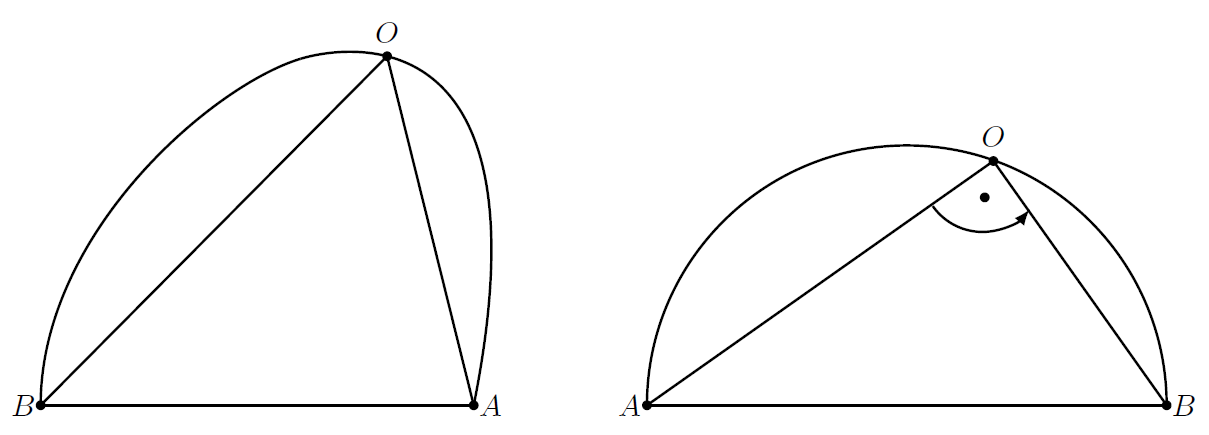Semicircle is cool.

At this point, the statement equivalent to the Steiner's conclusion remains to be proved — so how to determine in a different way that the semicircle is the searched curve? We will use here with other, simpler, isoperimetric problems, even considerations about the maximum area of a triangle with a given perimeter. The solution to this task is a rectangular triangle. So if our solution is to be a semicircle, then every angle inscribed in the arch should be a right angle. The closer the angle is to the angle of the right angle — the surface area increases. It is impossible not to mention the isoperimetric inequality that gave us dependence between perimeter of the circle with the candidate for the curve with the maximum area. If L is the perimeter of the circle, then the circle is equal to L2/4π, therefore the isoperimetric inequality between the area P and the perimeter of any closed curve is as follows:

P ≤ L2/4π

The equals sign is only for the circle.

# Edler's proof

Let us now turn to the Edler's proof, which supplements Steiner's proof of the existential part. Edler's procedure is a really brilliant jugglery of simpler isoperimetric statements. We now omit concave curves.
Let's consider any convex polygon ABCDE and use Steiner symmetrizaton on it.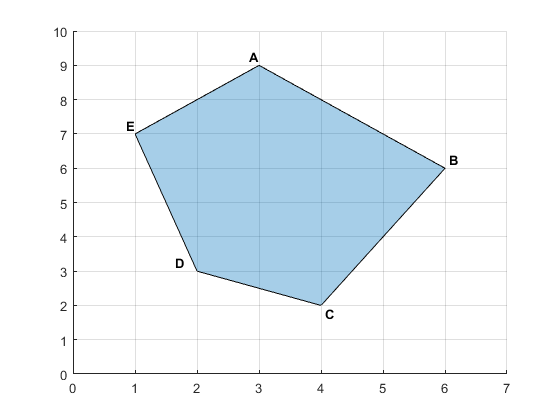Hello! I am arbitrary polygon. I am born in Matlab.

``````x1 = [1 ; 2 ; 4 ; 6 ; 3];
y1 = [7 ; 3 ; 2 ; 6 ; 9];

P1 = [x1 y1];
figure
polyin = polyshape(P1);
plot(polyin)
xlim([0 max(x1)+1])
ylim([0 max(y1)+1])
Area.a1 = polyarea(x1,y1);
Perimeter.a1 = perimeter(polyin);
grid on
---------------------------------------------------
Area =

struct with fields:

a1: 20.5000

Perimeter =

struct with fields:

a1: 17.9024
``````

Through its vertices we lead parallel to each other in a direction different from the polygon designated by the sides. We move point A along the line to any point A1. Through the A1 point we lead perpendicular to the already designated parallel ones. The sections lying inside the polygon are put on appropriate parallel lines so that they are symmetrical with respect to the straight line A1C1. In this way, we have received a new polygon with the same perimeter as the original but with a larger area.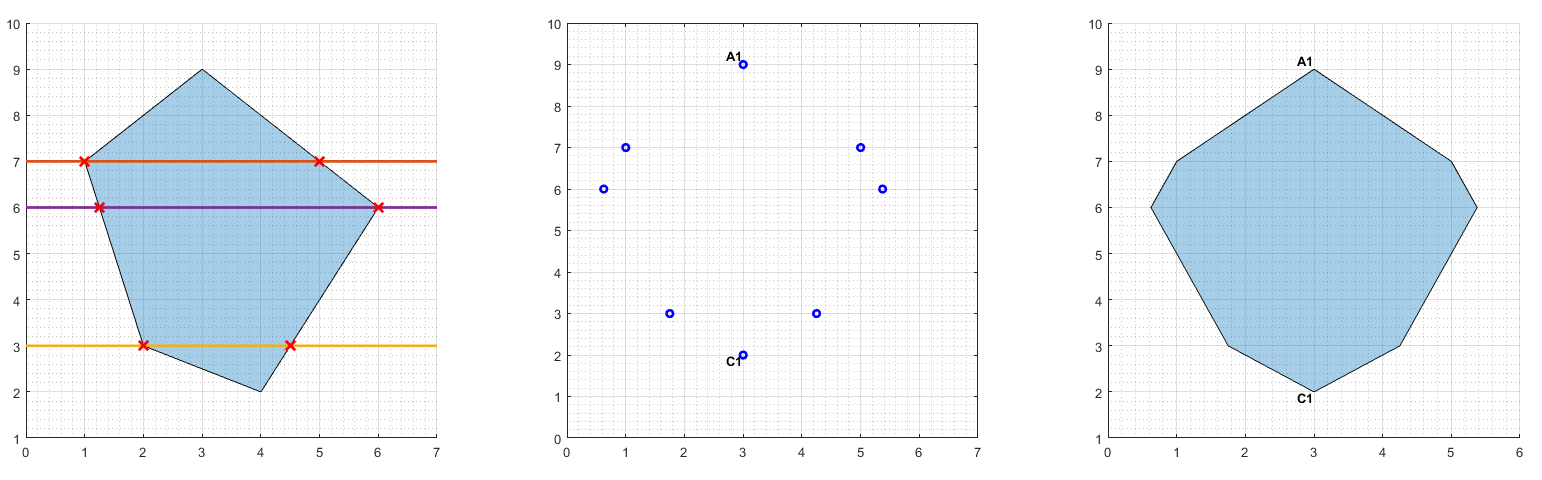First transformation.

We repeat this construction in the direction perpendicular to the straight A1C1. Again, we have a new polygon with the same perimeter and a larger area. The polygon has two mutually perpendicular axes of symmetry.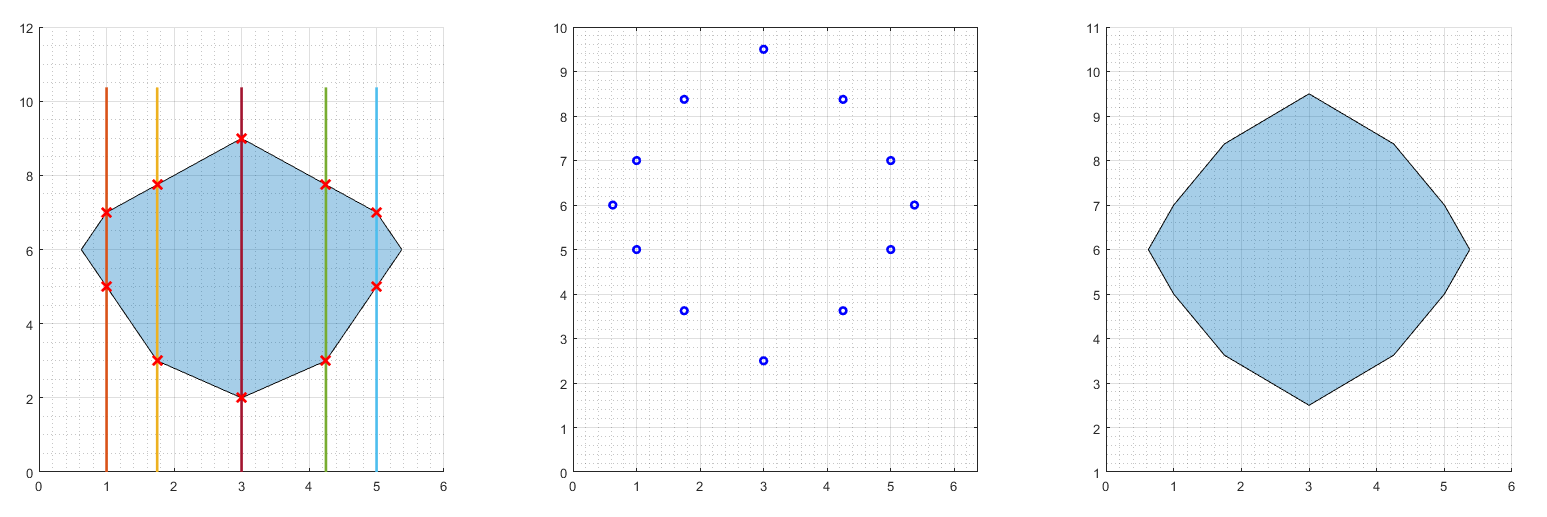Second transformation.

As you probably guessed — this construction can be repeated infinitely. This time, we take into account the quarter of our polygon and we will maximize its area. As a consequence, we will get a new polygon, the quarter of which will have the axis of symmetry contained in the arms of 1/4 pi. Repeating this construction infinitely, we get a polygon with the following number of symmetry axes:

0, 1, 2, 22, 23, 24, ...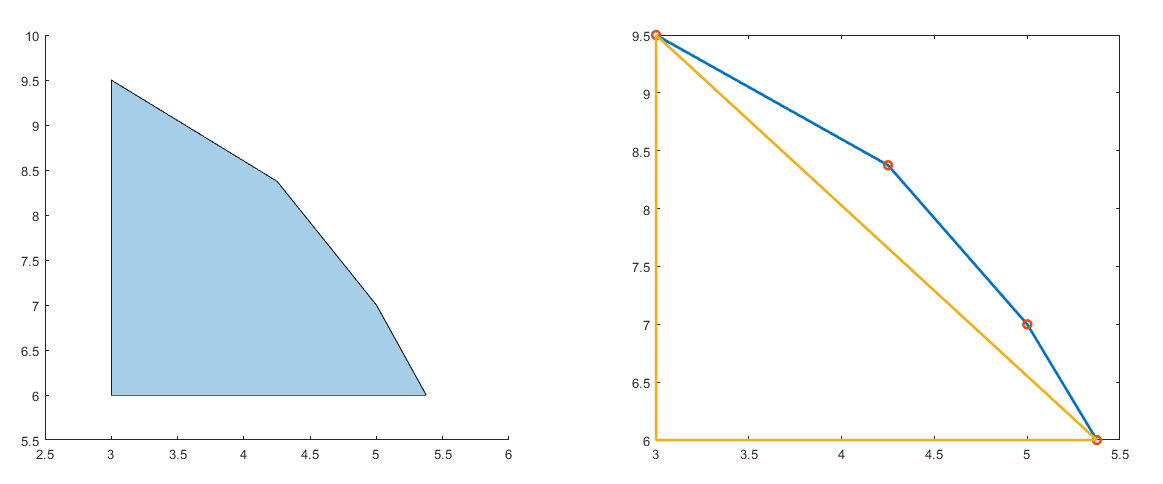Before third transformation.

After minor transformations resulting from the isoperimetry of the triangles, we obtain a regular polygon with an area greater than or equal to the input and a perimeter smaller or equal to the input one. From this it follows that of all n-sided polygons the largest area has a regular n-sided polygon.
The last point of consideration will be to prove that the circle has a larger area than the regular n-sided polygon with the same perimeter. I will try briefly to sketch the proof. We consider a circle and a regular n-sided polygon with the same perimeter. Next, we construct a new regular polygon described on the circle and a new circle inscribed in the input polygon. The ratio of the polygon perimeter to the circle perimeter is the same as the radius ratio of the new circle inscribed in the polygon to the radius of the input circle, and we know that this ratio is less than 1. Thus, the area of the circle is greater than the area of the regular polygon.
And at the end — I was curious how symmetrization works in practice and counted the perimeters and areas of the figures received. The results are as follows:

``````Area =

struct with fields:

a1: 20.5000                  % input
a2: 20.5000                  % 1st transformation
a3: 20.5000                  % 2nd transformation

>> Perimeter

Perimeter =

struct with fields:

a1: 17.9024                  % input
a2: 17.4024                  % 1st transformation
a3: 17.2638                  % 2nd transformation
``````

Task conditions are met — the area after transformation is not smaller than the input one, and the perimeter is not larger than the input one. Notabene, the isoareometric problem is very close to the isoperimetric problem.

# Summary

The Steiner constructions and all their derivative (evidence and theorems) are a very good geometrical exercise. In addition to specialized applications, eg in computer science and graph theory, mechanics, I could not find pristine applications of the isoperymetric problem. If anyone has any ideas I will be grateful. I think the number of applications is much larger than in the case of a paper clip ;).

References:
 Górnicki J.: Własności ekstremalne figur izoperymetrycznych na płaszczyźnie, from: Matematyka społeczeństwo Nauczanie, nr 5, Siedlce 1990.
 Courant R., Robbins H., Co to jest matematyka, Warszawa 1967.
 Tkocz T., Symetryzacja Steinera, DeltaMi, 04/2011.
 100 uses for paperclips

Photos/graphs:
 — Jess Watters, Unsplash

All figures are made in Texmaker and Matlab.